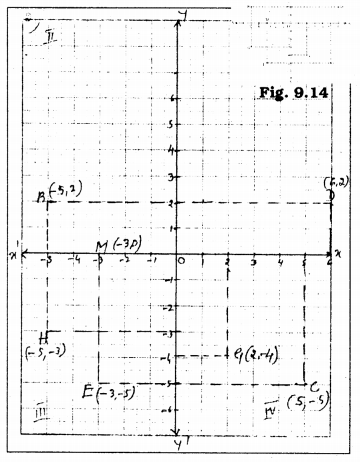# KSEEB Solutions for Class 9 Maths Chapter 9 Coordinate Geometry Ex 9.2

KSEEB Solutions for Class 9 Maths Chapter 9 Coordinate Geometry Ex 9.2 are part of KSEEB Solutions for Class 9 Maths. Here we have given Karnataka Board Class 9 Maths Chapter 9 Coordinate Geometry Exercise 9.2.

## Karnataka Board Class 9 Maths Chapter 9 Coordinate Geometry Ex 9.2

Question 1.
Write the answer of each of the following questions :
(i) What is the name of horizontal and the vertical lines drawn to determine the position of any point in the Cartesian plane ?
(ii) What is the name of each part of the plane formed by these two lines?
(iii) Write the name of the point where these two lines intersect.
Solution:
(i) In a Cartesian plane, to determine the position of any point, the horizontal line is called the x-axis. A vertical line is y-
The y-coordinate is also called the ordinate.
Horizontal line → xOx’
Vertical line → yOy’

(ii) The name of each part of the plane formed by these two lines is called Quadrant.

(iii) The name of the point where these two lines intersect is called the origin. Coordinates of origin are (0. 0).

Question 2.
See Fig.9.14, and write the following:Solution:

1. The coordinates of B : (-5, +2)
2. The coordinates of C. : (+5, -5)
3. The point identified by the coordinates (-3, -5) : E.
4. The point identified by the coordinates (2, -4) : G.
5. The abscissa of the point D : 6
6. The ordinate of the point H: -3
7. The coordinates of the point L : (0, +5)
8. The coordinates of the point M : (-3, 0).

We hope the KSEEB Solutions for Class 9 Maths Chapter 9 Coordinate Geometry Ex 9.2 helps you. If you have any query regarding Karnataka Board Class 9 Maths Chapter 9 Coordinate Geometry Exercise 9.2, drop a comment below and we will get back to you at the earliest.